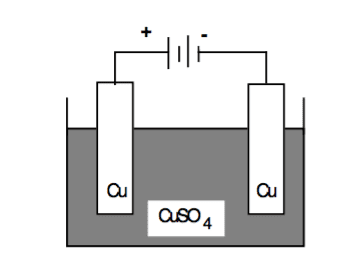# Solution molarity change in electrolytic cell

## Homework Statement

The diagram below shows an electrolysis cell which contains 1L of an aqueous 1M copper(II)sulfate solution.If 0.4 moles of electrons pass through the cell, the concentration of copper ions after passage of the charge will be

A) 0.4 M

B) 0.8 M

C) 1.0 M

D) 1.2M.

2. Homework Equations

## The Attempt at a Solution

I chose C because if 0.4 moles of electrons pass through the cell, then that means 0.2 moles of Cu2+ will be deposited into the solution from the anode and that means 0.2 moles of Cu2+ will be absorbed by the cathode. This gives no net change in molarity in the solution. Is this correct?

Cheers!

•